# Transfer Learning using PyTorch

Today we learn how to perform transfer learning for image classification using PyTorch.

# Introduction

## What is PyTorch?

PyTorch is an open-source machine learning library based on the Torch library, used for applications such as computer vision and natural language processing, primarily developed by Facebook’s AI Research lab (FAIR).

## What is Transfer Learning?

Transfer learning (TL) is a research problem in machine learning (ML) that focuses on storing knowledge gained while solving one problem and applying it to a different but related problem.

For example, the knowledge gained while learning to recognize cars could apply when trying to recognize trucks.

# Getting started

We use a Kaggle Notebook for this task since it provides free computation services which should be sufficient for the image classification task.

## Import Libraries

We import various libraries along with the submodules required for the image classification.

``````%matplotlib inline

# Import required libraries
import numpy as np
import pandas as pd
import time
import math
import tqdm as tqdm
import os
import csv
import torch
import torch.nn.functional as F
import torch.optim as optim
import torchvision.models as models
import matplotlib.pyplot as plt
from PIL import Image
from torch import nn
from torch.utils.data.dataset import Dataset
from torchvision import transforms, datasets

# Check if compute other than CPU is available
device = torch.device('cuda' if torch.cuda.is_available() else 'cpu')
``````

### Dataset

We use the 102 Category Flower Dataset for our image classification task. This dataset contains images of flowers belonging to 102 different categories.

### Set data folders

``````data_dir = '../input/oxford-flowers-data/flower_data'
train_dir = data_dir + '/train'
valid_dir = data_dir + '/valid'
test_dir = data_dir + '/test'
name_json = data_dir + '/cat_to_name.json'
``````

Here we have three main directories `train_dir`, `valid_dir`, and `test_dir` which contain training, validation, and testing data respectively.

We also have a `cat_to_name.json` file which contains the mapping of flower category to the name of the flower.

The dataset at hand contains training and validation images categorized inside their respective folders, however, the test images are not categorized and are merged in a single directory.

Thus, we need to define functions to load the test data as required by PyTorch during the training of the model.

``````def pil_loader(path):
with open(path, 'rb') as f:
img = Image.open(f)
return img.convert('RGB')
``````
``````class TestDataset(torch.utils.data.Dataset):
def __init__(self, path, transform=None):
self.path = path
self.files = []
for (dirpath, _, filenames) in os.walk(self.path):
for f in filenames:
if f.endswith('.jpg'):
p = {}
p['img_path'] = dirpath + '/' + f
self.files.append(p)
self.transform = transform

def __len__(self):
return len(self.files)

def __getitem__(self, idx):
img_path = self.files[idx]['img_path']
img_name = img_path.split('/')[-1]
if self.transform:
image = self.transform(image)
return image, 0, img_name
``````

### Label mapping

We load the labels JSON file and define a function to get the class/category of a flower by passing in an index.

``````import json

with open(name_json, 'r') as f:
``````
``````def get_cat_name(index):
return cat_to_name[idx_to_class[index]]
``````

### Transform

Now even though we might have enough data for training but performing various transforms or operations on the images helps the model learn better and perform efficiently on unseen data.

``````mean = [0.485, 0.456, 0.406]
std = [0.229, 0.224, 0.225]

normalize = transforms.Normalize(mean=mean, std=std)
data_transforms = transforms.Compose([
transforms.RandomRotation(10),
transforms.RandomResizedCrop(224),
transforms.RandomHorizontalFlip(),
transforms.ToTensor(),
normalize
])
test_transforms = transforms.Compose([
transforms.RandomResizedCrop(224),
transforms.ToTensor(),
normalize
])
``````

Next, we provide the image dataset directories and the respective transforms to the dataloader function which will actually load images as needed.

``````train_datasets = datasets.ImageFolder(train_dir, data_transforms)
val_datasets = datasets.ImageFolder(valid_dir, test_transforms)
test_datasets = TestDataset(test_dir, test_transforms)
``````
``````bs = 64

``````

Finally, we create a dictionary to get the class/category of a flower from an index.

``````idx_to_class = {val:key for key, val in val_datasets.class_to_idx.items()}
``````

### Plot images

Its always a good idea to take a look at the dataset before training the model. Hence, we define a `plot_img` function to plot a grid of random dataset images.

``````def plot_img(preds=None, is_pred=False):
fig = plt.figure(figsize=(8,8))
columns = 4
rows = 5

for i in range(1, columns*rows +1):

if is_pred:
img_xy = np.random.randint(len(test_datasets));
img = test_datasets[img_xy].numpy()
else:
img_xy = np.random.randint(len(train_datasets));
img = train_datasets[img_xy].numpy()

img = img.transpose((1, 2, 0))
img = std * img + mean

if is_pred:
plt.title(get_cat_name(preds[img_xy]) + "/" + get_cat_name(test_datasets[img_xy]))
else:
plt.title(str(get_cat_name(train_datasets[img_xy])))
plt.axis('off')
img = np.clip(img, 0, 1)
plt.imshow(img, interpolation='nearest')
plt.show()
``````
``````plot_img()
``````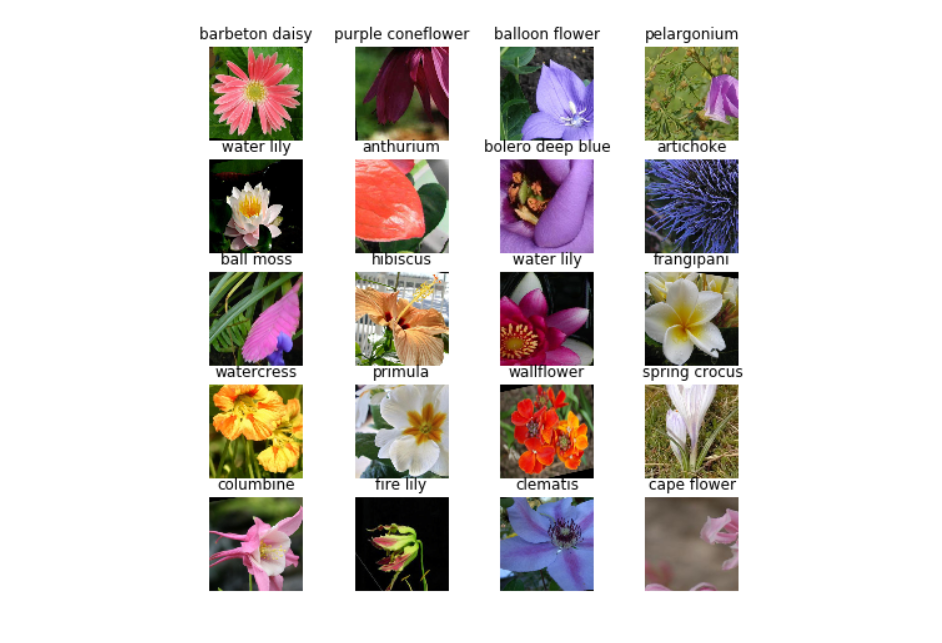## Helper functions

Next, we define helper functions which will be required later while training and evaluating the model.

A function that returns `accuracy` based on the output and target parameters.

``````def accuracy(output, target, is_test=False):
global total
global correct
batch_size = target.size(0)
total += batch_size
_, pred = output.max(dim=1)
if is_test:
preds.extend(pred)
correct += torch.sum(pred == target.data)
return  (correct.float()/total) * 100
``````

A `reset` function to reset/clear evaluation metrics.

``````def reset():
global total, correct
global train_loss, test_loss, best_acc
global trn_losses, trn_accs, val_losses, val_accs
total, correct = 0, 0
train_loss, test_loss, best_acc = 0.0, 0.0, 0.0
trn_losses, trn_accs, val_losses, val_accs = [], [], [], []
``````

A function to record the training and testing stats used to plot graphs.

``````class AvgStats(object):
def __init__(self):
self.reset()

def reset(self):
self.losses =[]
self.precs =[]
self.its = []

def append(self, loss, prec, it):
self.losses.append(loss)
self.precs.append(prec)
self.its.append(it)
``````

A function to save checkpoint if a new best score is achieved while training

``````def save_checkpoint(model, is_best, filename='./checkpoint.pth.tar'):
if is_best:
torch.save(model.state_dict(), filename)  # save checkpoint
else:
print ("=> Validation Accuracy did not improve")
``````

A function to load a saved checkpoint.

``````def load_checkpoint(model, filename = './checkpoint.pth.tar'):
sd = torch.load(filename, map_location=lambda storage, loc: storage)
names = set(model.state_dict().keys())
for n in list(sd.keys()):
if n not in names and n+'_raw' in names:
if n+'_raw' not in sd: sd[n+'_raw'] = sd[n]
del sd[n]
``````

## Cyclic Learning Rate

This method is described in the paper Cyclical Learning Rates for Training Neural Networks to find out the optimum learning rate.

The learning rate is increased from a lower value to a higher per iteration for some iterations till loss starts exploding. The learning rate one power lower than the one where loss is minimum is chosen as the optimum learning rate for training.

``````class CLR(object):
def __init__(self, optim, bn, base_lr=1e-7, max_lr=100):
self.base_lr = base_lr
self.max_lr = max_lr
self.optim = optim
self.bn = bn - 1
ratio = self.max_lr/self.base_lr
self.mult = ratio ** (1/self.bn)
self.best_loss = 1e9
self.iteration = 0
self.lrs = []
self.losses = []

def calc_lr(self, loss):
self.iteration +=1
if math.isnan(loss) or loss > 4 * self.best_loss:
return -1
if loss < self.best_loss and self.iteration > 1:
self.best_loss = loss

mult = self.mult ** self.iteration
lr = self.base_lr * mult

self.lrs.append(lr)
self.losses.append(loss)

return lr

def plot(self, start=10, end=-5):
plt.xlabel("Learning Rate")
plt.ylabel("Losses")
plt.plot(self.lrs[start:end], self.losses[start:end])
plt.xscale('log')

def plot_lr(self):
plt.xlabel("Iterations")
plt.ylabel("Learning Rate")
plt.plot(self.lrs)
plt.yscale('log')
``````

Here we implement Lookahead optimizer. As proposed, the Lookahead optimizer goes k steps forward and 1 step backward.

``````from torch.optim import Optimizer
from collections import defaultdict

def __init__(self, optimizer, alpha=0.5, k=5):
assert(0.0 <= alpha <= 1.0)
assert(k >= 1)
self.optimizer = optimizer
self.alpha = alpha
self.k = k
self.param_groups = self.optimizer.param_groups
self.state = defaultdict(dict)
for group in self.param_groups:
group['k_counter'] = 0
self.slow_weights = [[param.clone().detach() for param in group['params']] for group in self.param_groups]

def step(self, closure=None):
loss = self.optimizer.step(closure)
for group, slow_Weight in zip(self.param_groups, self.slow_weights):
group['k_counter'] += 1
if group['k_counter'] == self.k:
for param, weight in zip(group['params'], slow_Weight):
param.data.copy_(weight.data)
group['k_counter'] = 0

return loss

def state_dict(self):
fast_dict = self.optimizer.state_dict()
fast_state = fast_dict['state']
param_groups = fast_dict['param_groups']
slow_state = {(id(k) if isinstance(k, torch.Tensor) else k): v
for k, v in self.state.items()}
return {
'fast_state': fast_state,
'param_groups': param_groups,
'slow_state': slow_state
}

fast_dict = {
'state': state_dict['fast_state'],
'param_groups': state_dict['param_groups']
}
slow_dict = {
'state': state_dict['slow_state'],
'param_groups': state_dict['param_groups']
}
``````

## LR Finder

Now, we define the logic to find Learning Rate (LR) using CLR.

``````def update_lr(optimizer, lr):
for g in optimizer.param_groups:
g['lr'] = lr
``````
``````def lr_find(clr, model, optimizer=None):
running_loss = 0.
avg_beta = 0.98
model.train()
for i, (input, target) in enumerate(t):
input, target = input.to(device), target.to(device)
var_ip, var_tg = Variable(input), Variable(target)
output = model(var_ip)
loss = criterion(output, var_tg)

running_loss = avg_beta * running_loss + (1-avg_beta) *loss.item()
smoothed_loss = running_loss / (1 - avg_beta**(i+1))
t.set_postfix(loss=smoothed_loss)

lr = clr.calc_lr(smoothed_loss)
if lr == -1 :
break
update_lr(optimizer, lr)

# compute gradient and do SGD step
loss.backward()
optimizer.step()
``````

## Train and Test

We implement the train and test logic along with the fit method which will be used in the next step for model training.

``````def train(epoch=0, model=None, optimizer=None):
model.train()
global best_acc
global trn_accs, trn_losses
is_improving = True
counter = 0
running_loss = 0.
avg_beta = 0.98
for i, (input, target) in enumerate(trainloader):
bt_start = time.time()
input, target = input.to(device), target.to(device)
var_ip, var_tg = Variable(input), Variable(target)

output = model(var_ip)
loss = criterion(output, var_tg)

running_loss = avg_beta * running_loss + (1-avg_beta) *loss.item()
smoothed_loss = running_loss / (1 - avg_beta**(i+1))

trn_losses.append(smoothed_loss)

# measure accuracy and record loss
prec = accuracy(output.data, target)
trn_accs.append(prec)

train_stats.append(smoothed_loss, prec, time.time()-bt_start)
if prec > best_acc :
best_acc = prec
save_checkpoint(model, True)

# compute gradient and do SGD step
loss.backward()
optimizer.step()
``````
``````def test(model=None):
model.eval()
global val_accs, val_losses
running_loss = 0.
avg_beta = 0.98
for i, (input, target) in enumerate(validloader):
bt_start = time.time()
input, target = input.to(device), target.to(device)
var_ip, var_tg = Variable(input), Variable(target)
output = model(var_ip)
loss = criterion(output, var_tg)

running_loss = avg_beta * running_loss + (1-avg_beta) *loss.item()
smoothed_loss = running_loss / (1 - avg_beta**(i+1))

# measure accuracy and record loss
prec = accuracy(output.data, target, is_test=True)
test_stats.append(loss.item(), prec, time.time()-bt_start)

val_losses.append(smoothed_loss)
val_accs.append(prec)
``````
``````def fit(model=None, sched=None, optimizer=None):
print("Epoch\tTrn_loss\tVal_loss\tTrn_acc\t\tVal_acc")
for j in range(epoch):
train(epoch=j, model=model, optimizer=optimizer)
test(model)
if sched:
sched.step(j)
print("{}\t{:06.8f}\t{:06.8f}\t{:06.8f}\t{:06.8f}"
.format(j+1, trn_losses[-1], val_losses[-1], trn_accs[-1], val_accs[-1]))
``````

## Model and Training

### Initialize variables

We initialize all the variables that will be required later while training and evaluating the model.

``````train_loss = 0.0
test_loss = 0.0
best_acc = 0.0
trn_losses = []
trn_accs = []
val_losses = []
val_accs = []
total = 0
correct = 0
``````

### Model

We use a pretrained ResNet50, a variant of the ResNet model which has 48 Convolution layers along with 1 MaxPool and 1 Average Pool layer.

``````model = models.resnet50(pretrained=True)
``````

Next, we configure the last fully connected layer of the model and save the model checkpoint.

``````model.fc = nn.Linear(in_features=model.fc.in_features, out_features=102)

for param in model.parameters():

for param in model.fc.parameters():

model = model.to(device)
``````
``````save_checkpoint(model, True, 'before_start_resnet50.pth.tar')
``````

Define loss and optimizer, find learning rate, and plot a graph.

``````criterion = nn.CrossEntropyLoss()
optim = torch.optim.SGD(model.parameters(), lr=1e-2, momentum=0.9, weight_decay=1e-4)

``````
``````lr_find(clr, model, optim)
``````
``````clr.plot()
``````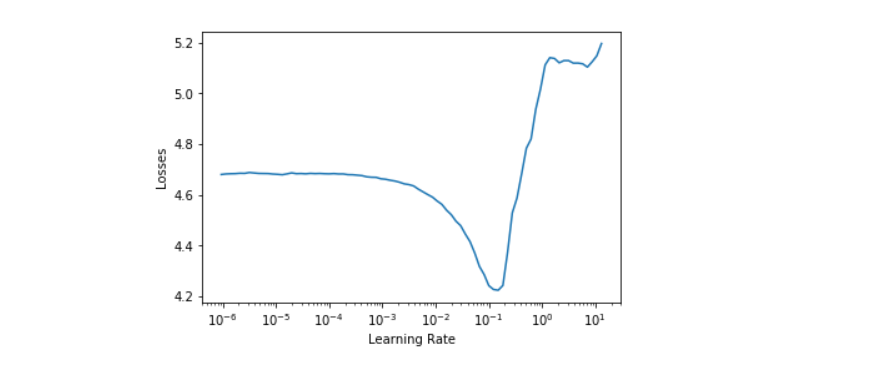### Training before unfreezing

Let’s load back the ResNet checkpoint that we saved earlier.

``````load_checkpoint(model, 'before_start_resnet50.pth.tar')
``````

Initialize parameters for the model training.

``````preds = []
epoch = 30
train_stats = AvgStats()
test_stats = AvgStats()
``````

We reset the parameters to makes sure that we record fresh results while training the model.

``````reset()
``````

Define loss and optimizer just like before.

``````criterion = nn.CrossEntropyLoss()
optim = torch.optim.SGD(model.parameters(), lr=1e-2, momentum=0.9, weight_decay=1e-4)
``````

Finally, train the model for 30 epochs.

``````fit(model=model, optimizer=optimizer)
``````

Save the model checkpoint after training.

``````save_checkpoint(model, True, 'before_unfreeze_resnet50.pth.tar')
``````

We plot training and validation loss as well as training and validation accuracy graphs to check the model performance.

``````plt.xlabel("Iterations")
plt.ylabel("Accuracy")
plt.plot(train_stats.precs, 'r', label='Train')
plt.legend()
``````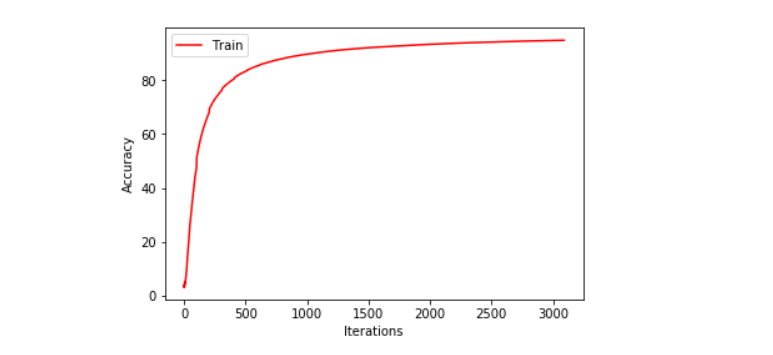``````plt.xlabel("Iterations")
plt.ylabel("Loss")
plt.plot(train_stats.losses, 'r', label='Train')
plt.legend()
``````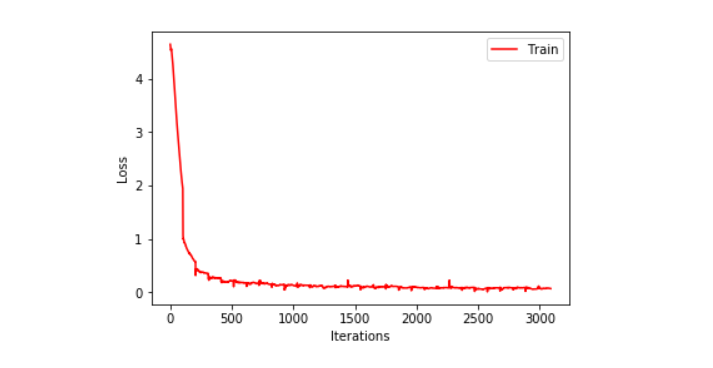``````plt.xlabel("Iterations")
plt.ylabel("Accuracy")
plt.plot(test_stats.precs, 'b', label='Valid')
plt.legend()
``````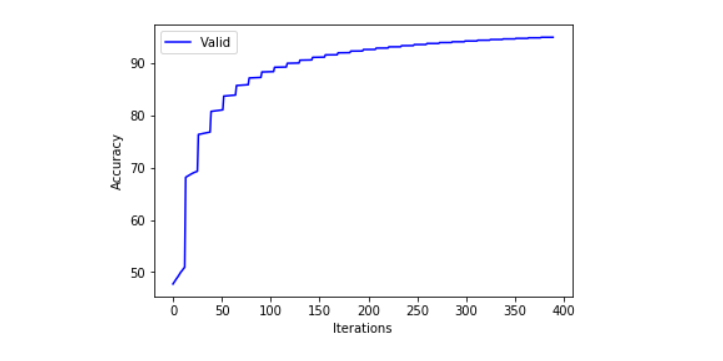``````plt.xlabel("Iterations")
plt.ylabel("Loss")
plt.plot(test_stats.losses, 'b', label='Valid')
plt.legend()
``````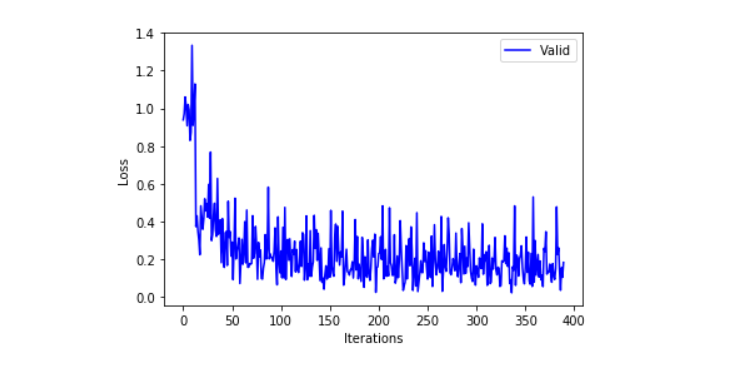### Training after unfreezing

Initialize parameters for the model training.

``````preds = []
train_stats = AvgStats()
test_stats = AvgStats()
``````

We reset the parameters to makes sure that we record fresh results while training the model.

``````reset()
``````

Enable differentiation tracking for each model parameter.

``````for param in model.parameters():
``````

Define the updated loss and optimizer and plot a losses vs learning rate graph.

``````optim = torch.optim.SGD(model.parameters(), lr=1e-6, momentum=0.9, weight_decay=1e-4)
clr = CLR(optim, len(trainloader), base_lr=1e-9, max_lr=10)
lr_find(clr, model, optim)
``````
``````clr.plot(start=0)
``````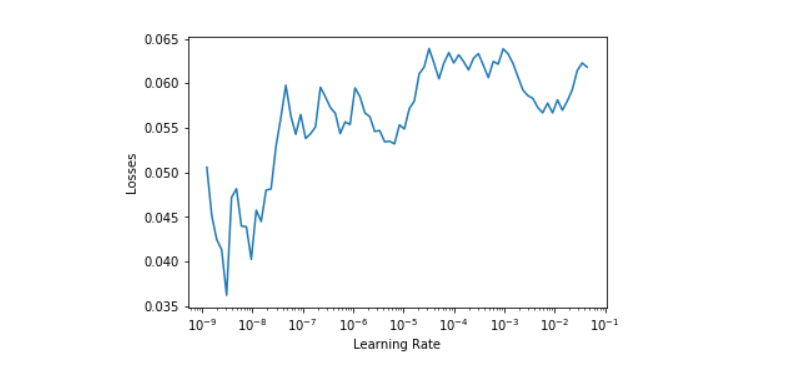Let’s load back the resnet checkpoint that we saved earlier.

``````load_checkpoint(model, 'before_unfreeze_resnet50.pth.tar')
``````

Initialize parameters for the model training.

``````preds = []
epoch = 10
train_stats = AvgStats()
test_stats = AvgStats()
``````

We reset the parameters to makes sure that we record fresh results while training the model.

``````reset()
``````

Define the updated learning rate and optimizer for training.

``````optim = torch.optim.SGD(model.parameters(), lr=1e-9, momentum=0.9, weight_decay=1e-4)
``````

At last, train the model for 10 epochs and save a checkpoint after training.

``````fit(model=model, optimizer=optimizer)
``````
``````save_checkpoint(model, True, 'after_unfreeze_resnet50.pth.tar')
``````

Next, we plot training and validation loss to compare the performance of the model.

``````plt.xlabel("Iterations")
plt.ylabel("Loss")
plt.plot(train_stats.losses, 'r', label='Train')
plt.legend()
``````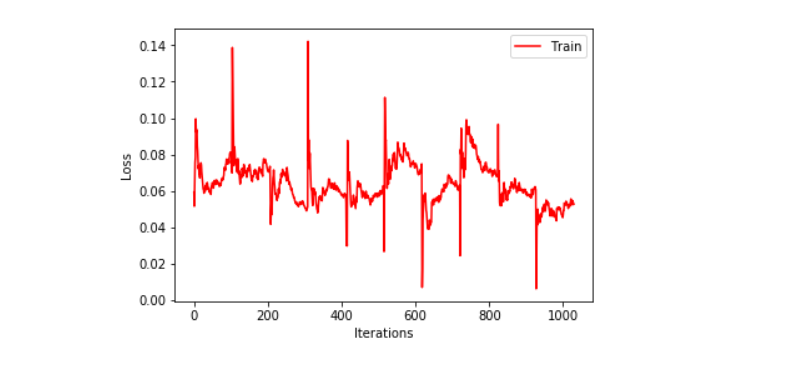``````plt.xlabel("Iterations")
plt.ylabel("Loss")
plt.plot(test_stats.losses, 'b', label='Valid')
plt.legend()
``````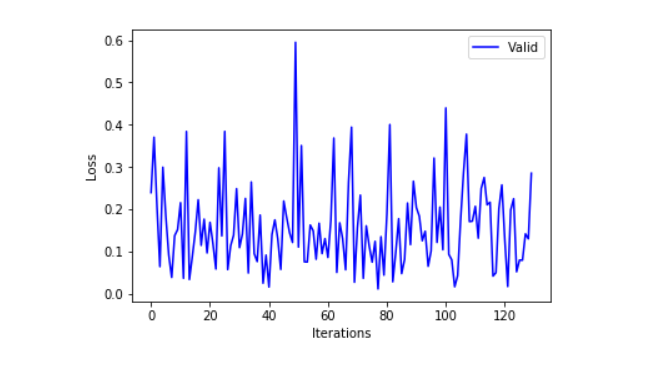## Testing

We define a `predict` method to predict labels for the test dataset.

``````def predict(img, model):
model.eval()
input = Variable(img)
input = input.to(device)
output = model(input)
_, pred = output.max(dim=1)
return pred.item()
``````

Predict labels or classes for all the images in the test dataset.

``````result = dict()
for i, (input, _, path) in enumerate(testloader):
predicted = predict(input, model)
result[path] = idx_to_class[predicted]
``````

Save the result for future reference.

``````csv_file = open('dict.csv', 'w')
writer = csv.writer(csv_file)
writer.writerow(['file_name','id'])
for key, value in result.items():
writer.writerow([key, value])
csv_file.close()
``````

Updated: